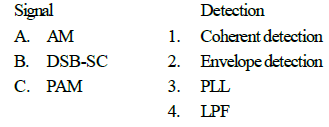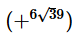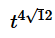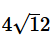# Test: Communication-1

## 25 Questions MCQ Test Basic Electronics Engineering for SSC JE (Technical) | Test: Communication-1

Description
Attempt Test: Communication-1 | 25 questions in 50 minutes | Mock test for Electrical Engineering (EE) preparation | Free important questions MCQ to study Basic Electronics Engineering for SSC JE (Technical) for Electrical Engineering (EE) Exam | Download free PDF with solutions
QUESTION: 1

Solution:
QUESTION: 2

Solution:
QUESTION: 3

### For coherent detection of digital signals, the receiver must be synchronised in

Solution:
QUESTION: 4

Solution:
QUESTION: 5Solution:
QUESTION: 6

Saving in power in DSB-SC system modulated at 80% is (in comparison with AM)

Solution:
QUESTION: 7

An amplifier has a power gain of 200. What is its gain in dB (log 102 = 0.3)

Solution:
QUESTION: 8

A DSB-SC signal is generated using the carrier cos (wct) and modulating signal x(t). The envelope of DSB -SC signal is

Solution:
QUESTION: 9

The waveform A cos (w1t + k cos w2t) is

Solution:
QUESTION: 10

The typical squelch circuit

Solution:
QUESTION: 11

Solution:
QUESTION: 12

An FM signal with modulation index mf is passed through a frequency tripler, the modulation index of output signal will be

Solution:
QUESTION: 13

An angle modulated signal is expressed by Fa(t)= cos (2 × 108t + 75 sin 2 × 103t). The peak frequency deviation of carrier is the

Solution:
QUESTION: 14

If we add the amplitude modulated signal and narrow band FM signal with same modulation index, than resultant signal will be

Solution:
QUESTION: 15

Which of the following modulation are used for telecasting TV programme

1. AM

2. FM

3. Pulse modulation

4. Phase modulation

Solution:
QUESTION: 16

Solution:
QUESTION: 17

In PCM system if the quantisation level are increased from 2 to 8, the relative bandwidth will be

Solution:
QUESTION: 18

The signal to quantisation noise ratio in an n-bit PCM system

Solution:
QUESTION: 19

In a delta modulation scheme, the step height is 75 mV and step size is 1.5 ms. The maximum slope that the staircase can track is

Solution:
QUESTION: 20

Quantisation noise occurs in

Solution:
QUESTION: 21

In case a signal band limited to fm is sampled at a rate less than 2fm, the constructed signal will be

Solution:
QUESTION: 22

In PCM if transmission path is very long

Solution:
QUESTION: 23

In a delta modulation system, granular noise occurs when the

Solution:
QUESTION: 24

Compander is used for

Solution:
QUESTION: 25

For 10 bit PCM system, the signal to quantiration noise ratio is 62 dB. If the number of bits are increased by 2, then signal to noise ratio will

Solution:Use Code STAYHOME200 and get INR 200 additional OFF Use Coupon Code